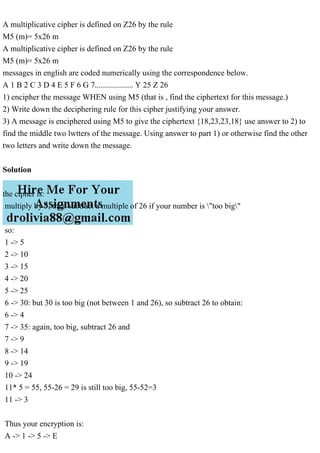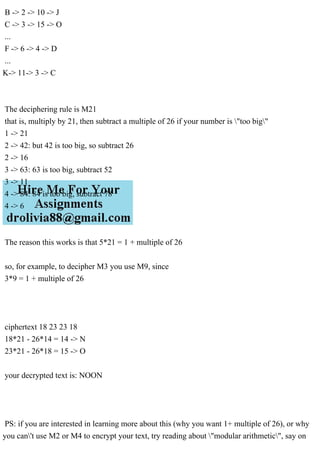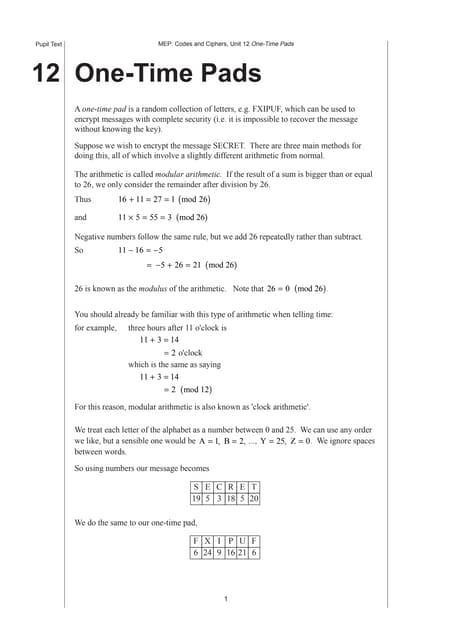Anúncio

# A multiplicative cipher is defined on Z26 by the ruleM5 (m)= 5x26 .pdf

amolsk22
2 de Apr de 2023Próximos SlideSharesOTP basic
Carregando em ... 3
1 de 3
Anúncio

### A multiplicative cipher is defined on Z26 by the ruleM5 (m)= 5x26 .pdf

1. A multiplicative cipher is defined on Z26 by the rule M5 (m)= 5x26 m A multiplicative cipher is defined on Z26 by the rule M5 (m)= 5x26 m messages in english are coded numerically using the correspondence below. A 1 B 2 C 3 D 4 E 5 F 6 G 7................... Y 25 Z 26 1) encipher the message WHEN using M5 (that is , find the ciphertext for this message.) 2) Write down the deciphering rule for this cipher justifying your answer. 3) A message is enciphered using M5 to give the ciphertext {18,23,23,18} use answer to 2) to find the middle two lwtters of the message. Using answer to part 1) or otherwise find the other two letters and write down the message. Solution the cipher is: multiply by 5, then subtract a multiple of 26 if your number is "too big" so: 1 -> 5 2 -> 10 3 -> 15 4 -> 20 5 -> 25 6 -> 30: but 30 is too big (not between 1 and 26), so subtract 26 to obtain: 6 -> 4 7 -> 35: again, too big, subtract 26 and 7 -> 9 8 -> 14 9 -> 19 10 -> 24 11* 5 = 55, 55-26 = 29 is still too big, 55-52=3 11 -> 3 Thus your encryption is: A -> 1 -> 5 -> E
2. B -> 2 -> 10 -> J C -> 3 -> 15 -> O ... F -> 6 -> 4 -> D ... K-> 11-> 3 -> C The deciphering rule is M21 that is, multiply by 21, then subtract a multiple of 26 if your number is "too big" 1 -> 21 2 -> 42: but 42 is too big, so subtract 26 2 -> 16 3 -> 63: 63 is too big, subtract 52 3 -> 11 4 -> 84: 84 is too big, subtract 78 4 -> 6 The reason this works is that 5*21 = 1 + multiple of 26 so, for example, to decipher M3 you use M9, since 3*9 = 1 + multiple of 26 ciphertext 18 23 23 18 18*21 - 26*14 = 14 -> N 23*21 - 26*18 = 15 -> O your decrypted text is: NOON PS: if you are interested in learning more about this (why you want 1+ multiple of 26), or why you can't use M2 or M4 to encrypt your text, try reading about "modular arithmetic", say on
3. wikipedia
Anúncio